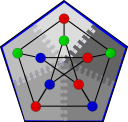#Boost C++ Libraries

...one of the most highly regarded and expertly designed C++ library projects in the world.

#R-MAT generator

``` template<typename RandomGenerator, typename Graph>
class rmat_iterator
{
public:
typedef std::input_iterator_tag iterator_category;
typedef std::pair<vertices_size_type, vertices_size_type> value_type;
typedef const value_type& reference;
typedef const value_type* pointer;
typedef void difference_type;

rmat_iterator();
rmat_iterator(RandomGenerator& gen, vertices_size_type n,
edges_size_type m, double a, double b, double c,
double d, bool permute_vertices = true);
// Iterator operations
reference operator*() const;
pointer operator->() const;
rmat_iterator& operator++();
rmat_iterator operator++(int);
bool operator==(const rmat_iterator& other) const;
bool operator!=(const rmat_iterator& other) const;
};
```

This class template implements a generator for R-MAT graphs [CZF04], suitable for initializing an adjacency_list or other graph structure with iterator-based initialization. An R-MAT graph has a scale-free distribution w.r.t. vertex degree and is implemented using Recursive-MATrix partitioning.

# Where Defined

<boost/graph/rmat_graph_generator.hpp>

# Constructors

```rmat_iterator();
```

Constructs a past-the-end iterator.

```rmat_iterator(RandomGenerator& gen, vertices_size_type n,
edges_size_type m, double a, double b, double c,
double d, bool permute_vertices = true);
```

Constructs an R-MAT generator iterator that creates a graph with n vertices and m edges. a, b, c, and d represent the probability that a generated edge is placed of each of the 4 quadrants of the partitioned adjacency matrix. Probabilities are drawn from the random number generator gen. Vertex indices are permuted to eliminate locality when permute_vertices is true.

# Example

```#include <boost/graph/adjacency_list.hpp>
#include <boost/graph/rmat_graph_generator.hpp>
#include <boost/random/linear_congruential.hpp>

typedef boost::rmat_iterator<boost::minstd_rand, Graph> RMATGen;

int main()
{
boost::minstd_rand gen;
// Create graph with 100 nodes and 400 edges
Graph g(RMATGen(gen, 100, 400, 0.57, 0.19, 0.19, 0.05), RMATGen(), 100);
return 0;
}
```

# Bibliography

 [CZF04] D Chakrabarti, Y Zhan, and C Faloutsos. R-MAT: A Recursive Model for Graph Mining. In Proceedings of 4th International Conference on Data Mining, pages 442--446, 2004.

Copyright (C) 2009 The Trustees of Indiana University.

Authors: Nick Edmonds and Andrew Lumsdaine